Solving Two-Step Linear Inequalities (single variable) MathBitsNotebook.com Terms of Use   Contact Person: Donna RobertsClick Basic Inequalities or Solving One Step Linear Inequalities for introductory information.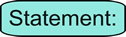If you can solve a linear equation, you can solve a linear inequality. The process is the same, with one important exception ...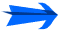... when you multiply (or divide) an inequality by a negative value, you must change the direction of the inequality.

Let's see why this "exception" is actually needed.

 We know that 3 is less than 7. Now, lets multiply both sides by -1. Examine the results (the products). ... written 3 < 7. ... written (-1)(3) ? (-1)(7) ... written -3 ? -7 On a number line, -3 is to the right of -7, making -3 greater than -7.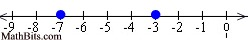-3 > -7 We have to reverse the direction of the inequality, when we multiply or divide by a negative value, in order to maintain a "true" statement.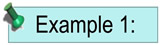Solve and graph the solution set of:   4x < 24 Proceed as you would when solving a linear equation such as 4x = 24: Divide both sides by 4. Note: The direction of the inequality stays the same since we did NOT divide by a negative value. Graph using an open circle for 6 (since x can not equal 6) and an arrow to the left (since our symbol is less than).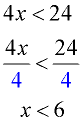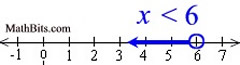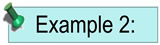Solve and graph the solution set of:   -5x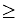25 Divide both sides by -5. Note: The direction of the inequality was reversed since we divided by a negative value. Graph using a closed circle for -5 (since x can equal -5) and an arrow to the left (since our final symbol is less than or equal to).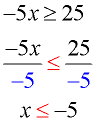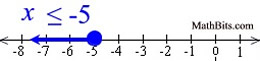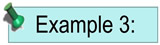Solve and graph the solution set of:   3x + 4 > 13 Proceed as you would when solving a linear equation such as 3x + 4 = 13: Subtract 4 from both sides. Divide both sides by 3. Note: The direction of the inequality stays the same since we did NOT divide by a negative value. Graph using an open circle for 3 (since x can not equal 3) and an arrow to the right (since our symbol is greater than).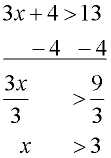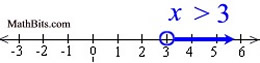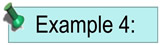Solve and graph the solution set of:   9 - 2x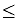3 Subtract 9 from both sides. Divide both sides by -2. Note: The direction of the inequality was reversed since we divided by a negative value. Graph using a closed circle for 3 (since x can equal 3) and an arrow to the right (since our symbol is greater than or equal to).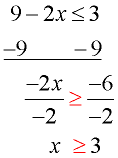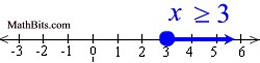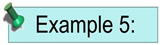Solve and graph the solution set of:   3 - 4x + 213 Combine 3 and 2 on the left side. Subtract 5 from both sides. Divide both sides by -4. Note: The direction of the inequality was reversed since we divided by a negative value. Graph using a closed circle for -2 (since x can equal -2).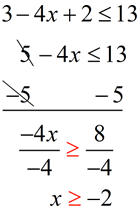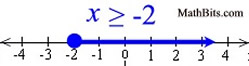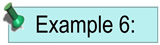Solve and graph the solution set of:   10 < 3(2x + 4) - 4x Distribute across parentheses. Combine the x-values. Subtract 12 from both sides. Divide both sides by 2. Note: There was no multiplication or division by a negative value, so the inequality symbol did not get reversed. Remember: -1 < x can be reversed so x is on the left side, and written as x > -1. Graph using a closed circle for -2 (since x can equal -2).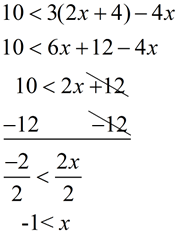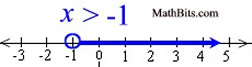Yes, there is a way to determine solutions for inequalities on your graphing calculator. Click the calculator at the right to see how to use the calculator with single variable inequalities.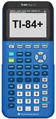For calculator help with inequalities (single variable) click here.